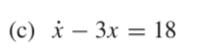# Identifying separable differential equations

#### Probability 0.99

##### New memberI am unsure about whether the differential equation above is separable or not. At first, I believed it was and tried solving the differential equation using the separation method, it gave me the wrong answer. I could only obtain the right answer using the integrating factor method.

Why is this equation not separable? It appears X can be on one side and the other is just the function of t...

I don't major in Maths, so I am not sure where I have gone wrong when deciding whether this equation is separable or not.

Thanks

Last edited:

#### MarkFL

##### Super Moderator
Staff member
Yes, the equation is separable...we may write it as:

$$\displaystyle \d{x}{t}=3x+18$$

or:

$$\displaystyle \frac{1}{x+6}\,dx=3\,dt$$

Integrate:

$$\displaystyle \int\frac{1}{x+6}\,dx=3\int\,dt$$

$$\displaystyle \ln|x+6|=3t+C$$

This implies:

$$\displaystyle x(t)=c_1e^{3t}-6$$

#### Probability 0.99

##### New member
Yes, the equation is separable...we may write it as:

$$\displaystyle \d{x}{t}=3x+18$$

or:

$$\displaystyle \frac{1}{x+6}\,dx=3\,dt$$

Integrate:

$$\displaystyle \int\frac{1}{x+6}\,dx=3\int\,dt$$

$$\displaystyle \ln|x+6|=3t+C$$

This implies:

$$\displaystyle x(t)=c_1e^{3t}-6$$
Thanks. I have noticed the mistake I have made now. Why did you change C to C1 at the end?

#### MarkFL

##### Super Moderator
Staff member
Thanks. I have noticed the mistake I have made now. Why did you change C to C1 at the end?
I mainly changed the parameter to show that it had been algebraically manipulated/changed. If we pick up here:

$$\displaystyle \ln|x+6|=3t+C$$

This of course directly implies:

$$\displaystyle x+6=\pm e^{3t+C}=\pm e^Ce^{3t}$$

Now, if we let:

$$\displaystyle c_1=\pm e^C$$

And consider that we lost the trivial solution:

$$\displaystyle x(t)\equiv-6$$

when we separated the variables, this means $$c_1\in\mathbb{R}$$, and we may state:

$$\displaystyle x+6=c_1e^{3t}$$

Hence:

$$\displaystyle x(t)=c_1e^{3t}-6$$

Treating the ODE as a linear equation, we avoid all this. Later, you will learn a method call undetermined coefficients that will allow you to solve linear equations even more efficiently. You will be able to see by inspection that the homogeneous solution is:

$$\displaystyle x_h(t)=c_1e^{3t}$$

And the particular solution must take the form:

$$\displaystyle x_p(t)=A\implies x_p'(t)=0$$ (here $$A$$ is a constant)

And by substitution into the ODE we find:

$$\displaystyle 0-3A=18\implies A=-6$$

Thus:

$$\displaystyle x_p(t)=-6$$

And, by the principle of superposition, we have the general solution:

$$\displaystyle x(t)=x_h(t)+x_p(t)=c_1e^{3t}-6\quad\checkmark$$

•Probability 0.99

#### Probability 0.99

##### New member
I mainly changed the parameter to show that it had been algebraically manipulated/changed. If we pick up here:

$$\displaystyle \ln|x+6|=3t+C$$

This of course directly implies:

$$\displaystyle x+6=\pm e^{3t+C}=\pm e^Ce^{3t}$$

Now, if we let:

$$\displaystyle c_1=\pm e^C$$

And consider that we lost the trivial solution:

$$\displaystyle x(t)\equiv-6$$

when we separated the variables, this means $$c_1\in\mathbb{R}$$, and we may state:

$$\displaystyle x+6=c_1e^{3t}$$

Hence:

$$\displaystyle x(t)=c_1e^{3t}-6$$

Treating the ODE as a linear equation, we avoid all this. Later, you will learn a method call undetermined coefficients that will allow you to solve linear equations even more efficiently. You will be able to see by inspection that the homogeneous solution is:

$$\displaystyle x_h(t)=c_1e^{3t}$$

And the particular solution must take the form:

$$\displaystyle x_p(t)=A\implies x_p'(t)=0$$ (here $$A$$ is a constant)

And by substitution into the ODE we find:

$$\displaystyle 0-3A=18\implies A=-6$$

Thus:

$$\displaystyle x_p(t)=-6$$

And, by the principle of superposition, we have the general solution:

$$\displaystyle x(t)=x_h(t)+x_p(t)=c_1e^{3t}-6\quad\checkmark$$
what does c1∈R mean? I major in Economics, sorry about my lack of knowledge. Thanks

#### MarkFL

##### Super Moderator
Staff member
what does c1∈R mean? I major in Economics, sorry about my lack of knowledge. Thanks
It's just shorthand to say that the parameter $$c_1$$ can be any real number (is an element of the reals $$\mathbb{R}$$), including zero.